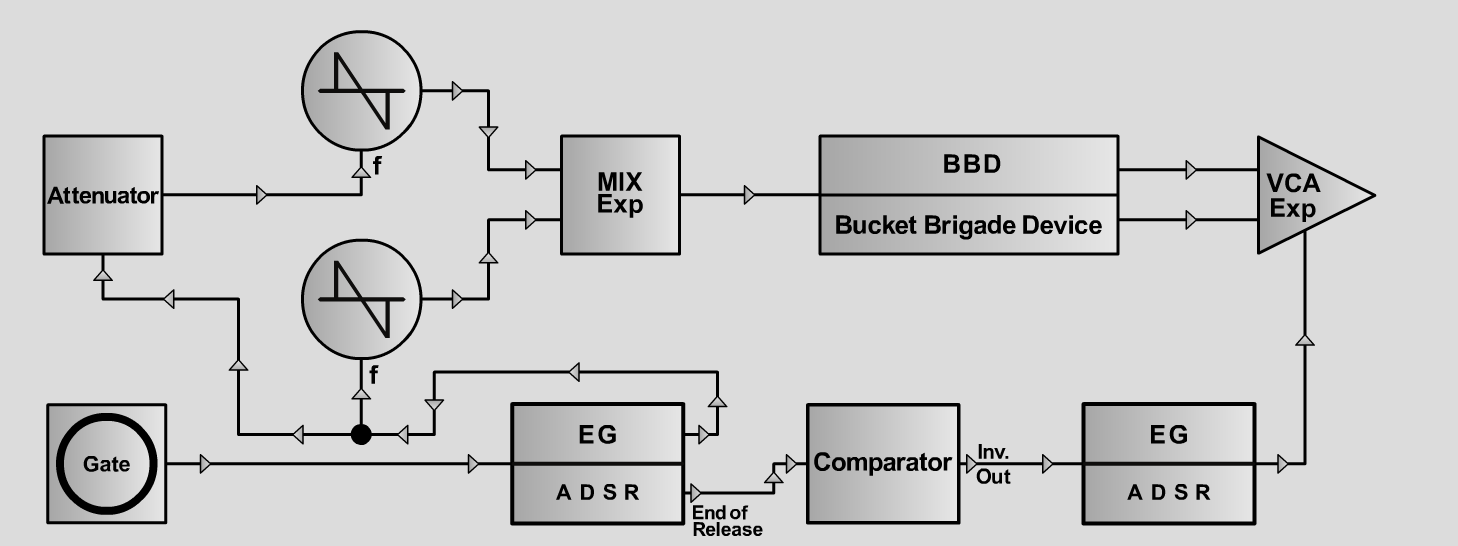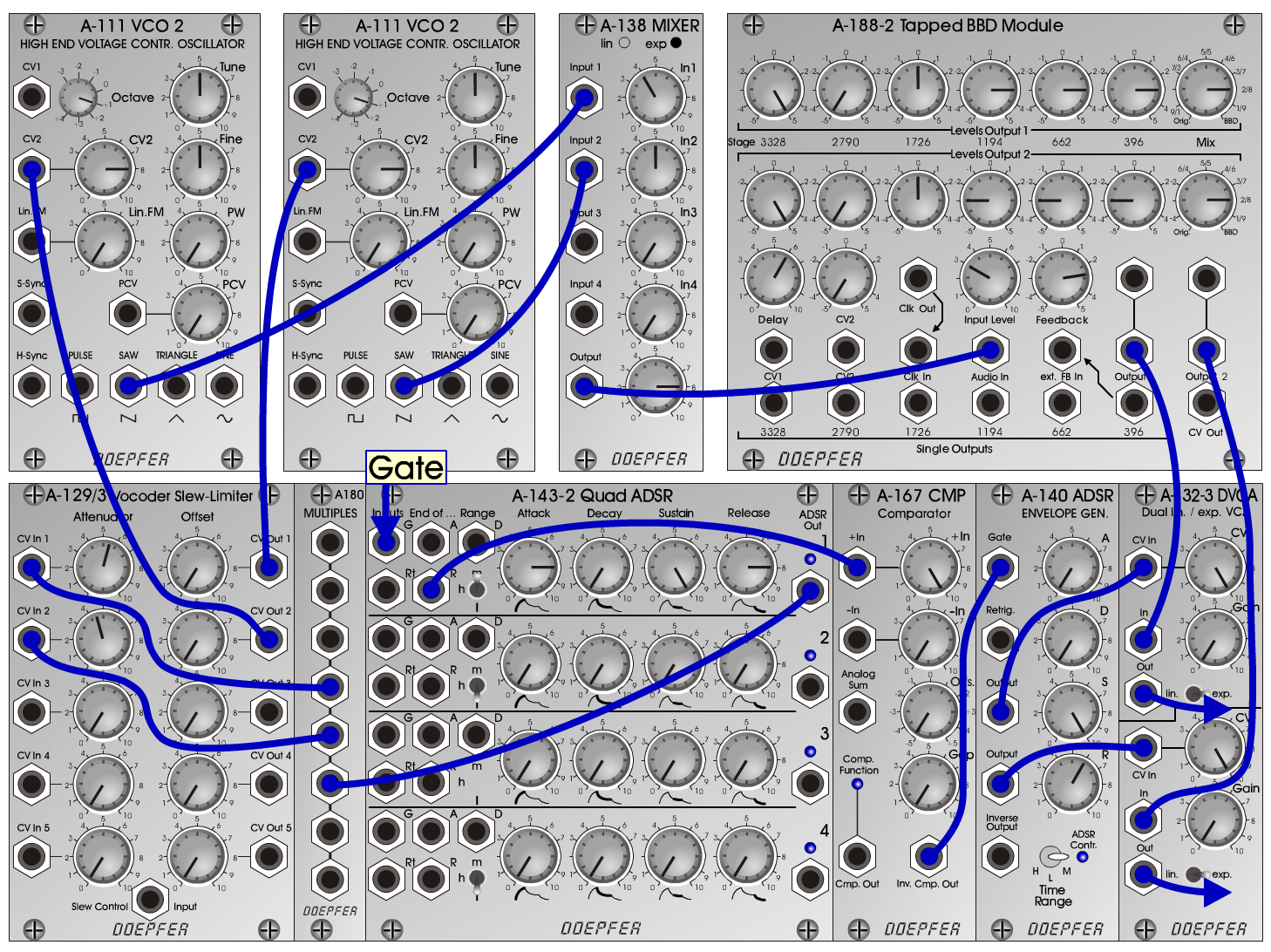Outer Space AlarmFXD o e p f e r A - 100 Connections: Settings: Outer Space Alarm 1: A-111/1 (Saw) <=> A-138 (Input 1)  A-111/2 (Saw) <=> A-138 (Input 2)  A-138 (Output) <=> A-188-2 (Audio In)  A-188-2 (Output 1) <=> 132-3/a (In)  A-188-2 (Output 2) <=> 132-3/b (In)  Gate <=> A-143-2/1 (G)  A-143-2/1 (ADSR Out) <=> A-180  A-180 <=> A-129/3 (CV In 1)  A-180 <=> A-129/3 (CV In 2)  A-129/3 (CV Out 1) <=> A-111/2 (CV2)  A-129/3 (CV Out 2) <=> A-111/1 (CV2)  A-143-2/1 (R) <=> A-167 (+ In)  A-167 (Inv. Cmp. Out) <=> A-140 (Gate)  A-140 (Output) <=> 132-3/a (CV In)  A-140 (Output) <=> 132-3/b (CV In)  132-3/a (Out) <=> Amp (left)  132-3/b (Out) <=> Amp (right) A-111/1 (Octave = +1, Tune = 5, Fine = 5, CV2 = 8)  A-111/2 (Octave = +1, Tune = 5, Fine = 5, CV2 = 8)  A-138 (In 1 = 4, In 2 = 5, Out = 8)  A-143-2 (Out 1: Range = m, Attack = 8, Decay = 0, Sustain = 10, Release = 8)  A-129/3 (Att 1 = 5.5, Offs 1 = 0, Att 2 = 4.5, Offs 2 = 0)  A-167 (+In = 10, -In = 0, Offs. = 0, Gap = 0)  A-140 (A = 0, D = 0, S = 10, R = 6, Range = M)  A-132-3 (above: CV = 10, Gain = 0, exp.; below: CV = 10, Gain = 0, lin.)  A-188-2 (Levels Output 1: 3328 = 5, 2790 = -5, 1726 = 0, 1194 = 3, 662 = 3, 396 = 3, Mix = 2/8,  Levels Output 2: 3328 = 5, 2790 = -5, 1726 = 0, 1194 = -3, 662 = -3, 396 = -3, Mix = 2/8,  Delay = 6, CV2 = 0, Input Level = 3, Feedback = 2.7) Outer Space Alarm 2: A-188-2 (Levels Output 1: 3328 = 5, 2790 = 4, 1726 = 3,  1194 = 2.5, 662 = 1.5, 396 = 1, Mix = 2/8;  Levels Output 2: 3328 = 1, 2790 = 1.5, 1726 = 2,  1194 = 3, 662 = 4, 396 = 5, Mix = 2/8;  Delay = 6, CV2 = 0, Input Level = 4, Feedback = 0) Outer Space Alarm 3: A-188-2 (Levels Output 1: 3328 = 5, 2790 = 5, 1726 = 3,  1194 = 2, 662 = 1, 396 = 1, Mix = 2/8;  Levels Output 2: 3328 = 5, 2790 = 5, 1726 = 3,  1194 = 2, 662 = 1, 396 = 1, Mix = 2/8;  Delay = 5, CV2 = 0, Input Level = 2.5, Feedback = -2) Outer Space Alarm 4: A-188-2 (Levels Output 1: 3328 = 5, 2790 = -5, 1726 = -5,  1194 = -5, 662 = -5, 396 =-5 , Mix = 1/9;  Levels Output 2: 3328 = -5, 2790 = 5, 1726 = 5,  1194 = 5, 662 = 5, 396 = 5, Mix = 1/9;  Delay = 8.5, CV2 = 0, Input Level = 2, Feedback = 3) Outer Space Alarm 5: (without BBD) A-188-2 (Levels Output 1: 3328 = 0, 2790 = 0, 1726 = 0,  1194 = 0, 662 = 0, 396 = 0, Mix = Orig.;  Levels Output 2: 3328 = 0, 2790 = 0, 1726 = 0,  1194 = 0, 662 = 0, 396 = 0, Mix = Orig.;  Delay = 0, CV2 = 0, Input Level = 6, Feedback = 0) Josef MuellerSound samples Outer Space Alarm 1 Outer Space Alarm 1 (reverb) Outer Space Alarm 2 Outer Space Alarm 2 (reverb) Outer Space Alarm 3 Outer Space Alarm 3 (reverb) Outer Space Alarm 4 Outer Space Alarm 4 (reverb) Outer Space Alarm 5 (without BBD) Outer Space Alarm 5 (reverb, without BBD)